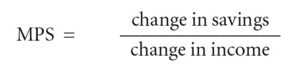marginal propensity to save

Also found in: Acronyms, Wikipedia.

marginal propensity to save (MPS)

the fraction of any change in NATIONAL INCOME that is saved (see SAVING):Alternatively, the change in savings can be expressed as a proportion of the change in DISPOSABLE INCOME. See PROPENSITY TO SAVE, MULTIPLIER.

References in periodicals archive ?
The marginal propensity to save (MIS) is found to be higher in the case of urban household.
For the Keynesian marginal propensity to save only the figure of .20 is used.
In short, SX will tend to markedly steeper than S'S' because its slope is the "marginal propensity to save out of transitory income," which can be quite high relative to the long-run propensity which is the slope of S'S'.
Signs of 44 and 45 are positive and negative respectively, if the marginal propensity to save, (1 - [C.sub.1]), is greater than the marginal propensity to invest out of income, I' ql (l/[F.sub.l]).
We have derived a more general conclusion in our synthesized model in that although the initial trade restriction policy will create a trade surplus to boost the GDP, whether dNX > 0 or < 0 will depend upon whether the marginal propensity to save exceeds the marginal propensity to invest, i.e., [alpha] > [gamma] or [alpha] < [gamma].
Even though foreign capital has not been productive, the marginal propensity to save seems sufficient to permit SSA to generate a surplus for debt service.
(3) Next we introduce slope dummies to see a shift in marginal propensity to save (MPS).
The marginal propensity to save (MPS), calculated at the mean value of household income, is found to vary from 0.21 to 0.23 in the case of the urban area, and from 0.30 to 0.37 in the case of the rural area, depending on the choice of the functional form.
In addition, it is shown that the marginal propensity to save out of anticipated labour income is positive under quite general conditions.

Site: Follow: Share:
Open / Close